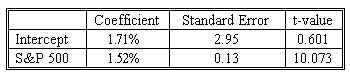### CFA Practice Question

There are 208 practice questions for this study session.

### CFA Practice Question

To help gain a better understanding of the relationship between the return on the common stocks of small companies and the return on the S&P 500 Index, you run a simple linear regression to quantify this relationship, using the monthly return on small stocks as the dependent variable and the monthly return on the S & P 500 as the independent variable. The results of the regression are shown below:The t-statistic critical level at the 0.01 level is 2.66
Residual Standard Error is 19.85
Correlation coefficient is 0.7740
N=75
F-value = 101.465 on 1,730 degrees of freedom.
The percent of the variation in the return on the dependent variable (return on small stocks) explained by the return on the independent variable (return on the S & P 500 ) for the period under study was:
A. 10.07 percent.
B. 59.91 percent.
C. 77.40 percent.
Explanation: Coefficient of determination = (correlation coefficient)2
R2= 0.7742
=0.5991